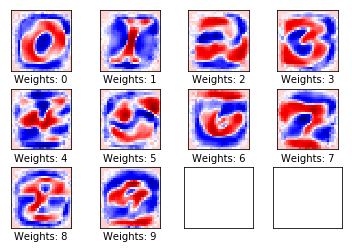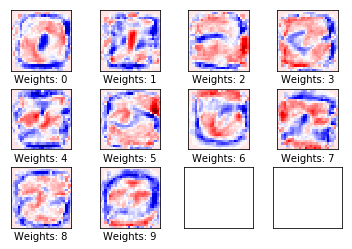# visualize weights (Tensorflow)

For this example, we would be using a function that displays the weights of trained model.  By plotting the model weights you can visualize each digit filter that the model was trained to recognize. We use weights placeholder variable that to make this possible. I will be using dataset MIST.</p>

### Start Tensorflow training session

import time

sess = tf.Session(config=tf.ConfigProto(log_device_placement=True))
sess.run(tf.global_variables_initializer())

def train():
beginTime = time.time()
for offset in range(, len(X_train), BATCH_SIZE):
end = offset + BATCH_SIZE
batch_x, batch_y = X_train[offset:end], y_train[offset:end]
sess.run(training_operation, feed_dict={x: batch_x, y: batch_y})

validation_accuracy = evaluate(X_validation, y_validation, sess)
endTime = time.time()
print ("Total time {:5.2f}s accuracy:{}".format(endTime - beginTime, validation_accuracy))


### plot_weights() is a helper-function that would plot the neuron weights

As you plug the weights back into an image you can see one for each digit that the model is trained to recognize. Individual weights represent the strength of connections between units. If the weight from class 1 to unit 2 has a greater magnitude (all else being equal), it means that A has greater influence over B.

w = sess.run(weights)

"weights" is a TensorFlow variable we initialize at the begging of our code. For this example "weights" were initialized with zeros as a 2-dimensional tensor with img_size_flat rows and num_classes columns. Because we still have the training session open we can access it after one EPOCH and see how much it was changed.

#Let’s view the weights as a 28x28 grid where the weights are arranged exactly like their corresponding pixels.
def plot_weights():
# Get the values for the weights from the TensorFlow variable.
w = sess.run(weights)
# Get the lowest and highest values for the weights.
# This is used to correct the colour intensity across
# the images so they can be compared with each other.
w_min = np.min(w)
w_max = np.max(w)
print(w_min)
print(w_max)
# Create figure with 3x4 sub-plots,
# where the last 2 sub-plots are unused.
fig, axes = plt.subplots(3, 4)
fig.subplots_adjust(hspace=0.3, wspace=0.3)

for i, ax in enumerate(axes.flat):
# Only use the weights for the first 10 sub-plots.
if i<10:
# Get the weights for the i'th digit and reshape it.
# Note that w.shape == (img_size_flat, 10)
image = w[:, i].reshape(img_shape)

# Set the label for the sub-plot.
ax.set_xlabel("Weights: {0}".format(i))

# Plot the image.
ax.imshow(image, vmin=w_min, vmax=w_max, cmap='seismic')

# Remove ticks from each sub-plot.
ax.set_xticks([])
ax.set_yticks([])

# Ensure the plot is shown correctly with multiple plots
# in a single Notebook cell.
plt.show()

This is how our weights look after one EPOCH
train()
plot_weights()


Total time  0.40s accuracy:0.897
-0.245477
0.206278### How does weight change with more training?

Early units receive weighted connections from input pixels. The activation of each unit is a weighted sum of pixel intensity values, passed through an activation function. Because the activation function is monotonic, a given unit's activation will be higher when the input pixels are similar to the incoming weights of that unit (in the sense of having a large dot product). So, you can think of the weights as a set of filter coefficients, defining an image feature. For units in higher layers (in a feedforward network), the inputs aren't from pixels anymore, but from units in lower layers. So, the incoming weights are more like 'preferred input patterns'.

In :
for i in range (10):
train()
plot_weights()


Total time  0.40s accuracy:0.9104
Total time  0.37s accuracy:0.9158
Total time  0.37s accuracy:0.9184
Total time  0.37s accuracy:0.9204
Total time  0.37s accuracy:0.9226
Total time  0.37s accuracy:0.924
Total time  0.37s accuracy:0.9248
Total time  0.37s accuracy:0.9264
Total time  0.37s accuracy:0.926
Total time  0.37s accuracy:0.926
-1.1957
1.07317

This is how our weights look after ten EPOCH## Manuel Cuevas

Hello, I'm Manuel Cuevas a Software Engineer with background in machine learning and artificial intelligence.